# Kerala Plus Two Maths Important Questions

Most students studying in the plus two are well aware of the significance of Class 12 Maths in their life. Since they are well aware of how crucial the year is for them, students are on the hunt to find as many resources or study material as they can, so that they can prepare effectively for the exams. BYJU’s come to the help of these students by compiling here the Kerala Plus Two Maths Important Questions.

These questions are useful for students as it covers almost all the main topics and concepts of the subject. It gives the students an overview of the type of questions that are normally asked in the exams. Students who have prepared from this also feel quite confident to write the exam, as they are more acquainted with the exam pattern.

## KBPE Class 12th Maths Important Questions & Answers

Question 1: If f (x) = x / (x – 1), x ≠ 1,

[a] Find f o f (x)

[b] Find the inverse of f

Solution:

[a] f o f (x) = f ( f (x))

= f (x / x – 1)

= (x / x – 1) / [(x / x – 1) – 1]

= x / [x – (x – 1)]

= x

[b] y = x / (x – 1)

xy – x = y

xy – y = x

y (x – 1) = x

f -1 (y) = y / (y – 1)

f -1 (x) = x / (x – 1)

Question 2[a]: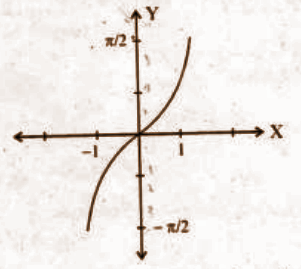Identify the function from the above graph.

[i] tan-1 x [ii] sin-1 x [iii] cos-1 x [iv] cosec-1 x

[b] Find the domain and range of the function represented by the graph.

Solution:

Domain → [-1, 1]

Range → [- 𝛑 / 2, 𝛑 / 2]

[c] Prove that tan-1 (1 / 2) + tan-1 (2 / 11) = tan-1 (3 / 4).

Solution:

tan-1 x + tan-1 y = tan-1 [(x + y) / (1 – xy)]

tan-1 (1 / 2) + tan-1 (2 / 11) = tan-1 [(1 / 2 + 2 / 11) / (1 – [(1 / 2 * 2 / 11)]]

= tan-1 (15 / 20)

= tan-1 (3 / 4)

Question 3: Consider the following figure: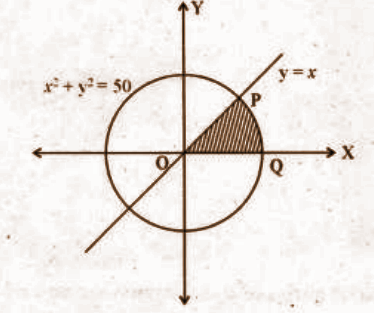[a] Find the point of intersection P of the circle x2 + y2 = 50 and the line y = x.

Solution:

x2 + y2 = 50

x2 + x2 = 50

2x2 = 50

x2 = 50 / 2

x = √25

x = ± 5

y = ± 5

The point of intersection is P (5, 5).

[b] Find the area of the shaded region.

Solution:

Required area = ∫05 x dx + ∫5√50 √50 – x2 dx

= (x2 / 2)05 + [(x / 2) √50 – x2 + (50 / 2) sin-1 (x / √50)]

= 25 / 2 + 25𝛑 / 2 – (25 / 2) – (25𝛑 / 4)

= 25𝛑 / 4

Question 4[a]: Prove that for any vectors a, b, c, [a + b, b + c, c + a] = 2 [a . b . c].

Solution:

[a + b, b + c, c + a] = (a + b) . [(b + c) x (c + a)]

= (a + b) . [b x c + b x a + c x c + c x a]

= (a + b) . [b x c + b x a + c x a]

= a . (b x c) + a . (b x a) + a . (c x a) + b . (b x c) + b . (b x a) + b . (c x a)

= 2 [a . b . c]

[b] Show that if [a + b, b + c, c + a] are coplanar, then a, b, c are also coplanar.

Solution:

[a b c] = 0

Hence a, b, c are also coplanar.

Question 5[a]: Find the equation of a plane which makes x, y, z intercepts respectively as 1, 2, 3.

Solution:

x / 1 = y / 2 = z / 3 = 1

6x + 3y + 2z = 6

[b] Find the equation of a plane passing through the point (1, 2, 3) which is parallel to the above plane.

Solution:

A plane parallel to the given plane is 6x + 3y + 2z = k.

Since it passes through (1, 2, 3), k = 18.

The equation of the plane is 6x + 3y + 2z = 18.

Question 6[a]: Prove that the function defined by f (x) = cos (x2) is a continuous function.

Solution:

f (x) = cos x

g (x) = x2

Both are continuous functions.

Composition of two continuous functions is continuous.

f (g (x)) = f o g (x) = cos (x2) is continuous.

[b] [i]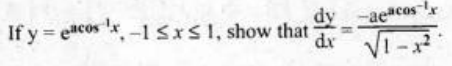[ii] Hence prove that (1 – x2) d2y / dx2 – x (dy / dx) – a2y = 0.

Solution:

[i]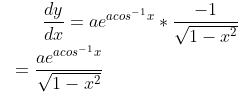[ii] (√1 – x2) dy / dx = -ay

(1 – x2) (dy / dx)2 = a2y2

(1 – x2) 2 (dy / dx) * (d2y / dx2) + (dy / dx)2 * (2x) = 2a2y (dy / dx)

(1 – x2) (d2y / dx2) – x (dy / dx) – a2y = 0

Question 7[a]: Find x and y if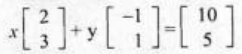.

Solution:

2x – y = 10 —- (1)

3x + y = 5 —- (2)

_________

5x = 15

x = 15 / 5

x = 3

Substitute the value of x in (1),

2 * 3 – y = 10

6 – y = 10

6 – 10 = y

y = – 4

So, x = 3 and y = -4.

[b] Express the matrix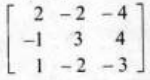as a sum of symmetric and skew-symmetric matrices.

Solution: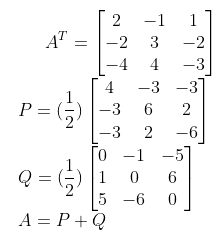Question 8:

[a] ∫sin mx dx

[b] ∫1 dx / √x2 + 2x + 2

[c] ∫x dx / (x + 1) (x + 2)

Solution:

[a] ∫sin mx dx

= (- cos mx / m) + c

[b] x2 + 2x + 2 = (x + 1)2 + 1

∫dx / √x2 + 2x + 2 = ∫dx / √(x + 1)2 + 1

= log [(x + 1)] + √x2 + 2x + 2 + c

[c] ∫x dx / (x + 1) (x + 2)

x / (x + 1) (x + 2) = A / (x + 1) + B / (x + 2)

A = -1, B = 2

∫x dx / (x + 1) (x + 2) = ∫(-1 / (x + 1) dx + ∫2 / (x + 2) dx

= – log (x + 1) + 2 log (x + 2) + c

Question 9[a]: Find the angle between the lines x – 2 / 2 = y – 1 / 5 = z + 3 / -3 and x + 2 / -1 = y – 4 / 8 = z – 5 / 4.

[b] Find the shortest distance between the pair of lines.

r = (i + 2j + 3k) + ƛ [i – 3j + 2k]

r = (4i + 5j + 6k) + 𝛍 [2i + 3j + k]

Solution:

a1 = 2, b1 = 5, c1 = -3 and a2 = -1, b2 = 8, c2 = 4

cos θ = a1a2 + b1b2 + c1c2 / √a12 + b12 + c12 * √a22 + b22 + c22

= – 2 + 40 – 12 / √4 + 25 + 9 √1 + 64 + 16

= 26 / 9√38

θ = cos-1 [26 / 9√38] [b] a1 = i + 2j + 3k, b1 = i – 3j + 2k

a2 = 4i + 5j + 6k, b2 = 2i + 3j + k

Shortest distance = |(a2 – a1) . (b1 x b2) / |b1 x b2||

(a2 – a1) = 3i + 3j + 3k

(b1 x b2) = – 9i + 3j + 9k

Shortest distance = [- 27 + 9 + 27] / √171

= 3 / √19

Question 10[a]: Let R be a relation defined on A = {1, 2, 3} by R = [{1, 3} , {3, 1}, {2, 2}]. R is

(a) Reflexive (b) Symmetric

(c) Transitive (d) Reflexive but not transitive

[b] Find f o g and g o f if f(x) = |x + 1| and g (x) = 2x – 1.

Solution:

f o g (x) = f (g (x)) = f (2x – 1)

= |2x – 1 + 1|

= |2x|

g o f (x) = g (f (x)) = g (x + 1)

= 2 |x + 1| – 1

[c] Let “*” be a binary operation defined on N x N by (a, b) * (c, d) = (a + c, b + d). Find the identity element for * if it exists.

Solution:

If (c, d) is the identity element,

(a, b) * (c, d) = (a, b)

(a, b) * (c, d) = (a + c, b + d)

(a + c, b + d) = (a, b)

(c, d) = (0, 0) is not an element of N x N.

So, the identity element doesn’t exist.

Question 11[a]: Consider the linear programming problem:

Maximise Z = 50x + 40y

Subject to constraints

x + 2y ≥ 10

3x + 4y ≤ 24

x ≥ 0, y ≥ 0

[a] Find the feasible region.

[b] Find the corner points of the feasible region.

[c] Find the maximum value of Z.

Solution:

[a]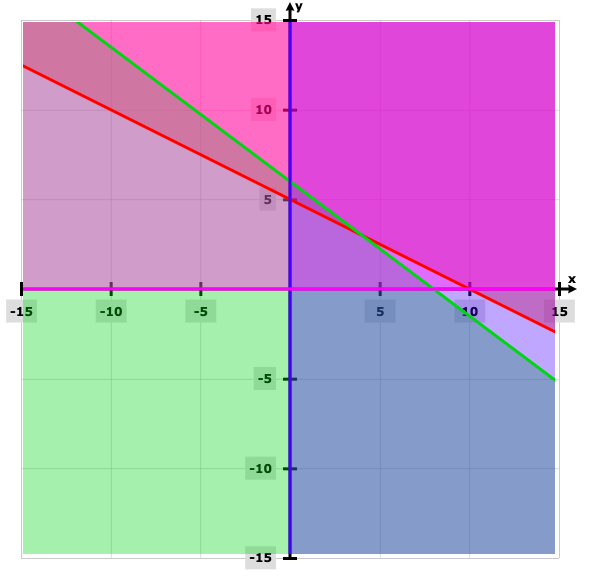[b] The corner points are (0, 5), (0, 6), (4, 3).

[c] At (0, 5), Z = 200

(0, 6), Z = 240

(4, 3), Z = 320

Z is maximum at (4, 3) and it is 320.

Question 12[a]: The angle between the vectors i + j and j + k is

(a) 60o (b) 30o (c) 45o (d) 90o

[b] If a, b, c are unit vectors such that a + b + c = 0, find the value of a . b + b . c + c . a.

Solution:

[a] Answer: [a] [b] |a x b x c| = 0

(a + b + c)2 = 0

|a|2 + |b|2 + |c|2 + 2ab + 2bc + 2ca = 0

3 + 2 (a . b + a . c + b . c) = 0

(a . b + a . c + b . c) = – 3 / 2

Question 13[a]: If A =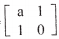such that A2 = I then a equals to

(a) 1 (b) -1 (c) 0 (d) 2

[b] Solve the system of equations:

x – y + z = 4

2x + y – 3z = 0

x + y + z = 2 by matrix method.

Solution: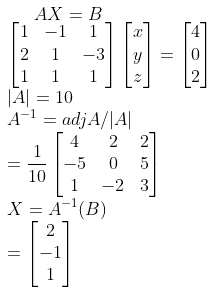Question 14[a]: Area bounded by the curves y = cos x, x = 𝛑 / 2, x = 0, y = 0 is

(a) 1 / 2 (b) 2 / 𝛑 (c) 1 (d) 𝛑 / 2

[b] Find the area between the curve y2 = 4ax and x2 = 4ay, a > 0.

Solution:

[b]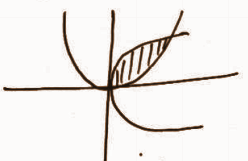The point of intersection is (4a, 4a).

Area = ∫04a √4ax dx – ∫04a (x2 / 4a) dx

= 2√a [(x3/2 / (3 / 2)]04a – (1 / 4a) (x3 / 3)04a

= [16 / 3] a2

Question 15[a]: The function f:N → N, given by f (x) = 2x is

(a) one-one but onto (b) one-one but not onto

(c) not one-one not onto (d) onto but not one-one

[b]: Find g o f (x), if f (x) = 8x3 and g (x) = x1/3.

Solution:

g o f (x) = g (f ( x))

= g (8x3)

= (8x3)

= 2x

[c]: Let * be an operation such that a * b = LCM of a and b defined on the set A = {1, 2, 3, 4, 5}. Is * a binary operation? Justify your answer.

Solution:

a * b = LCM of a and b

a, b ∈ {1, 2, 3, 4, 5}

Let a = 2, b = 3

a * b = 2 * 3 = LCM of 2 and 3 is 6

Since the element 6 doesn’t exist in the set {1, 2, 3, 4, 5}, * is not a binary operation.

Question 16[a]: Prove that ∫cos2 x dx = (x / 2) + (sin 2x / 4) + c.

Solution:

cos2 x = (1 + cos 2x) / 2

∫cos2 x dx = (1 / 2) ∫(1 + cos 2x) dx

= (1 / 2) [x + (sin 2x / 2)] dx

[b] Find ∫dx / √2x – x2.

Solution:

2x – x2 = – (x2 – 2x)

= – (x2 – 2x + 1 – 1)

= – [(x – 1)2 – 12]

= 1 – (x – 1)2

∫dx / √2x – x2 = ∫dx / √1 – (x – 1)2

= sin-1 (x – 1) + c

[c] Find ∫x cos x dx.

Solution:

∫x cos x dx

= x sin x – ∫ sin x dx

= x sin x + cos x + c

Question 17[a]: Find the shortest distance between the lines r = i + j + ƛ (2i – j + k) and r = 2i + j – k + 𝛍 (3i – 5j + 2k).

Solution:

Shortest distance = |[(a2 – a1) . (b1 – b2)] / |[b1 x b2]||

a1 = i + j

a2 = 2i + j – k

b1 = 2i – j + k

b2 = 3i – 5j + 2k

(a2 – a1) = i – k

[b1 x b2] = 3i – j + 7k

|[b1 x b2]| = √59

Shortest distance = 10 / √59

Question 18[a]: Equation of the plane with intercepts 2, 3, 4 on the x, y and z-axis respectively is

(i) 2x + 3y + 4z = 1 (ii) 2x + 3y + 4z = 12

(iii) 6x + 4y + 3z = 1 (iv) 6x + 4y + 3z = 12

Solution: [iv]

[b] Find the cartesian equation of the plane passing through the points A (2, 5, -3), B (- 2, – 3, 5) and C (5, 3, – 3).

Solution:

The equation is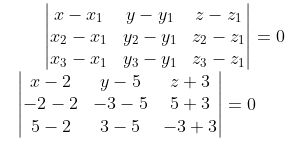(x – 2) 16 + (y – 5) 24 + (z + 3) 32 = 0

2x + 3y + 4z = 7

Question 19[a]: If P (A) = 0.3, P (B) = 0.4, then the value of P (A ⋃ B) where A and B are independent events is

(i) 0.48 (ii) 0.51 (iii) 0.52 (iv) 0.58

Solution: [iv]

[b] A card from a pack of 52 cards is lost. From the remaining cards of the pack, 2 cards are drawn and are found to be diamonds. Find the probability of the lost card being a diamond.

Solution:

E1: The lost card is a diamond.

E2: The lost card is not a diamond.

A: Selecting 2 diamonds from the remaining cards

P (E1) = 13 / 52 = 1 / 4

P (E2) = 39 / 52 = 3 / 4

P (A / E1) = 12C2 / 51C2 = 12 * 11 / 51 * 50

P (A / E2) = 13C2 / 51C2 = 13 * 12 / 51 * 50

P (E1 / A) = [P (E1) * P (A / E1)] / [P (E1) * P (A / E1)] + [P (E2) * P (A / E2)]

= [(1 / 4) * [12 * 11 / 51 * 50]] / [(1 / 4) * (12 * 11 / 51 * 50) + (3 / 4) * (13 * 12 / 51 * 50)]

= 132 / 600

= 11 / 50

Question 20: A pair of dice is thrown 4 times. If getting a doublet is considered as a success,

[i] Find the probability of getting a doublet.

[ii] Find the probability of two successes.

Solution:

[i] 1 / 6

[ii] n = 4

q = 1 – p

= 1 – (1 / 6)

= 5 / 6

P (X = 2) = 4C2 (5 / 6)2 (1 / 6)2

= 25 / 216

Question 21[a]: Choose the correct statement related to matrices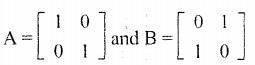(i) A3 = A, B3 ≠ 0 (ii) A3 ≠ A, B3 = B

(iii) A3 = A, B3 = B (iv) A3 ≠ A, B3 ≠ B

[b] If M =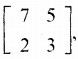then verify the equation M2 – 10M + 11I2 = 0.

Solution: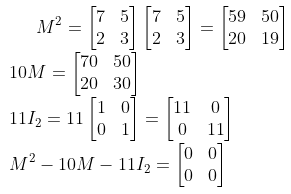[c] Inverse of the matrix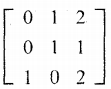.

Solution: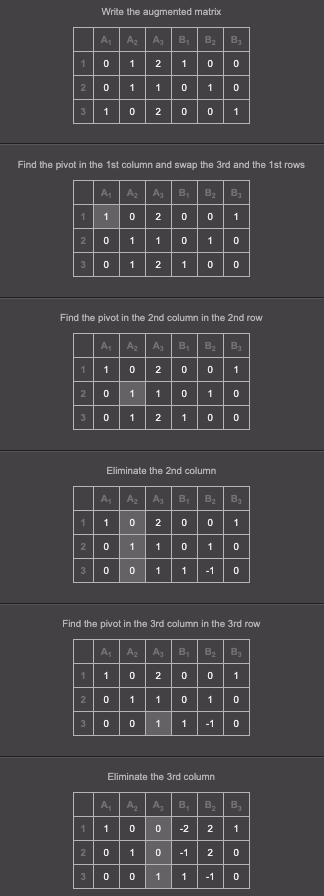There is the inverse matrix on the right.

Question 22[a]: Prove that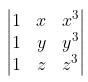= (x + y + z) (x – y) (y – z) (z – x).

OR

[b] Prove that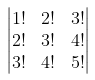= 4!.

Solution:

[a]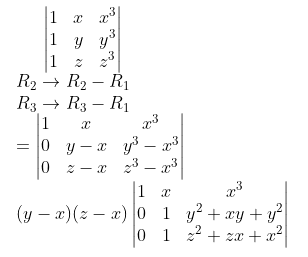= (y – x) (z – x) [(z2 + zx + x2) – (y2 + xy + x2)]

= (y – x) (z – x) [z2 – y2 + x (z – y)]

= (y – x) (z – x) [(z + y) (z – y) + x (z – y)]

= (y – x) (z – x) [(z – y) (x + y + z)]

= (x + y + z) (x – y) (y – z) (z – x)

OR

[b]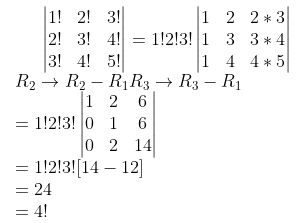Question 23[a]: Find a and b if the function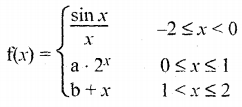is a continuous function on [-2, 2].

Solution:

[a] f (x) is continuous at x = 0

lim x→0- f (x) = lim x→0+ f (x)

lim x→0 sin x / x = limx→0 (a * 2x)

1 = a * 20

a = 1

f (x) is continuous at x = 1

lim x→1- f (x) = lim x→1+ f (x)

lim x→1- (a * 2x) = lim x→1+ b + x

a * (21) = b + 1

2a = b + 1

2 = b + 1

b = 1

[b] How many of the functions f (x) = |x|, g (x) = |x|2 and h(x) = |x|3 are not differentiable at x = 0?

(i) 0 (ii) 1 (iii) 2 (iv) 3

Question 24[a]: What is the value of ∫01 x (1 – x)9 dx?

(i) 1 / 10 (ii) 1 / 11 (iii) 1 / 90 (iv) 1 / 110

[b] Find ∫01 (2x + 3) dx as the limit of a sum.

Solution:

01 (2x + 3) dx

Let f (x) = 2x + 3

a = 0, b = 1, nh = b – a = 1 – 0 = 1

h = (b – a) / n = 1 – 0 / n = 1 / n

= (b – a) lim n→∞ [1 / n] [f (a) + f (a + h) + f (a + 2h) …… f (a + [n – 1]h)

= (1) lim n→∞ [1 / n] [f (0) + f (h) + f (2h) + ….. f ([n – 1]h]

= lim n→∞ [1 / n] [3 + f (1 / n) + f (2 * (1 / n)) + f [(n – 1) * (1 / n)]

= lim n→∞ [1 / n] [3 + [(2 / n) + 3] + [(4 / n) + 3] + …… [2 (n – 1) / n + 3]]

= lim n→∞ n (1 / n) {(3 + 3 + 3 + ….n times) + [(2 / n) + (4 / n) + ….+ 2(n – 1) / n]}

= lim n→∞ (1 / n) [3n + (2 / n) (1 + 2 + … + n – 1)]

= lim n→∞ (1 / n) [3n + (2 / n) (n – 1)(n – 1 + 1) / 2]

= lim n→∞ (1 / n) [3n + (2 / n) (n – 1) (n / 2)]

= lim n→∞ (1 / n) [3n + n – 1]

= lim n→∞ (1 / n) (4n – 1)

= lim n→∞ (n / n) [4 – 1 / n]

= lim n→∞ [4 – 1] / n

= 4 – 0

= 4

Question 25[a]: Write the Cartesian equation of the straight line through the point (1, 2, 3) and along the vector 3i + j + 2k.

(b) Write a general point on this straight line.

(c) Find the point of intersection of this straight line with the plane 2x + 3y – z + 2 = 0.

(d) Find the distance from (1, 2, 3) to the plane 2x + 3y – z + 2 = 0.

Solution:

[a] Equation of a line passing through a point (x1, y1, z1) and parallel to a line with direction cosines a, b, c is (x – x1) / a = (y – y1) / b = (z – z1) / c

Since line passes through (1, 2, 3),

x1 = 1, y1 = 2, z1 = 3

Also, the line is parallel to 3i + j + 2k.

a = 3, b = 1, c = 2

Equation of the line in cartesian form is given by

(x – 1) / 3 = (y – 2) / 1 = (z – 3) / 2

[b] Let (x – 1) / 3 = (y – 2) / 1 = (z – 3) / 2 = k

x = 3k + 1

y = k + 2

z = 2k + 3 is a general point on this straight line [here k is a constant].

[c] The equation of the plane is 2x + 3y − z + 2 = 0

Substituting the general point in the equation of the plane gives

⇒ 2 (3k + 1) + 3 (k + 2) − (2k + 3) + 2 = 0

⇒ k = −1

∴ The point of intersection is (−2,1,1).

[d] The direction cosines of the line and plane are (3, 1, 2) and (2, 3, −1).

∴ The angle between the line and the plane is

(3, 1, 2) . (2, 3, −1) = |(3, 1, 2)| × |(2, 3, −1)| cosα

cos α = 1 / 2

Distance between the points (1, 2, 3) and (−2, 1, 1) is √14 units.

sin α = (perpendicular distance from the plane) / √14

The perpendicular distance from the plane is √21 / 2 units.

Question 26: A random variable X has the following distribution function :

 X 0 1 2 3 4 P (x) k 3k 5k 7k 4k

(i) Find k.

(ii) Find the mean and the variance of the random variable x.

[i] ∑Pi = 1

k + 3k + 5k + 7k + 4k = 1

20k = 1

k = 1 / 20

[ii]
 X 0 1 2 3 4 P (x) 1 / 20 3 / 20 5 / 20 7 / 20 4 / 20 X * P(x) 0 3 / 20 10 / 20 21 / 20 16 / 20 X2 * P(x) 0 3 / 20 20 / 20 63 / 20 64 / 20

Mean = ∑ x * P(x) = 50 / 20 = 5 / 2

Variance = ∑ x2 * P(x) – [∑ x * P(x)]2 = 5 / 4

Question 27[a]: Write the function whose graph is shown below.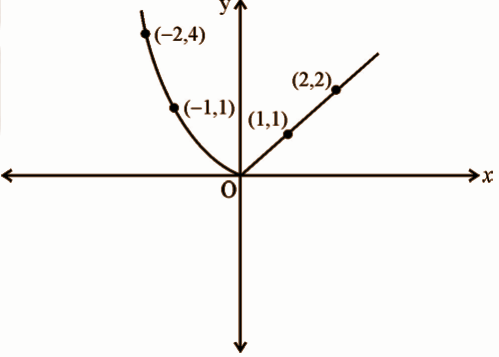(b) Discuss the continuity of the function obtained in part (a).

(c) Discuss the differentiability of the function obtained in part (a).

Solution:

[a] f (x) =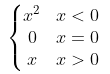[b] lim x→0+ f (x) = lim x→0+ (x) = 0

lim x→0- f (x) = lim x→0- (x2) = 0

f (0) = 0

f (x) is continuous at x = 0.

[c] f (x) is not differentiable at x = 0.

Question 28: A cuboid with a square base and given volume ‘V’ is shown in the figure.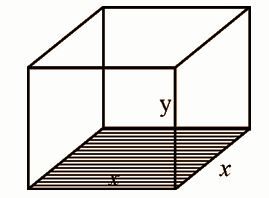(a) Express the surface area ‘s’ as a function of x.

(b) Show that the surface area is minimum when it is a cube.

Solution:

[a] Surface area (S) = 2x2 + 4xy

v = x2y

= 2x2 + 4x * (v / x2)

= 2x2 + (4v / x)

[b] ds / dx = 4x – (4v / x2)

ds / dx = 0

v = x3

x = (v)

d2s / dx2 = 4 + (8v / x3) = 4 + 8 = 12 > 0

Hence, S is minimum.

y = v / x2 = x3 / x2 = x

Therefore, cuboid becomes a cube.

Question 29: The position vectors of three points A, B, C are given to be i + 3 j + 3k, 4i + 4k and –2 i + 4 j + 2k respectively.

(a) Find AB and AC.

(b) Find the angle between AB and AC.

(c) Find a vector which is perpendicular to both AB and AC having a magnitude 9 units.

Solution:

[a] AB = 3i – 3j + k

BC = – 3i + j – k

[b] |AB| = √19

|AC| = √11

cos θ = |[AB . AC] / [|AB| . |AC|]

= |(- 13) / [√19 . √11]|

= 13 / [√19 . √11]

θ = cos-1 [13 / [√19 . √11]] [c] n = (AB x AC) / |(AB x AC)|

= 2i – 6k

|AB x AC| = √40

The required vector = 9 (2i – 6k) / √40

Question 30[a]: Write all the direction cosines of the x-axis.

(b) If a line makes angles ɑ, β, 𝝲 with x, y, z axes respectively, then prove that sin2 ɑ + sin2 β + sin2 𝝲 = 2.

(c) If a line makes equal angles with the three coordinate axes, find the direction cosines of the lines.

Solution:

[a] 1, 0, 0 or cos 0, cos 90, cos 90

[b] cos2 ɑ + cos2 β + cos2 𝝲 = 1

(1 – sin2 ɑ) + (1 – sin2 β) + (1 – sin2 𝝲) = 1

sin2 ɑ + sin2 β + sin2 𝝲 = 2

[c] ɑ = β = 𝝲

cos ɑ = cos β = cos 𝝲

cos2 ɑ + cos2 β + cos2 𝝲 = 1

3 cos2 ɑ = 1

cos ɑ = 1 / √3

Similarly, β = 1 / √3 and 𝝲 = 1 / √3